output.to from Sideway
Draft for Information Only

# Engineering Mechanic Static

1. Engineering Mechanics Statics

Engineering Mechanics Statics is about forces in static equilibrium.
http://output.to/sideway/default.asp?qno=110100001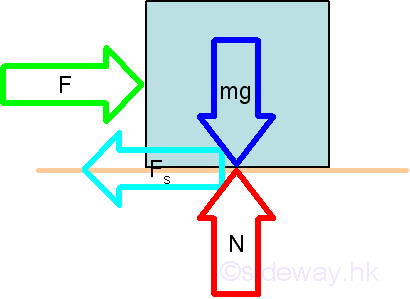2. Force is the resultant of interaction between two objects in the form of magnitude and direction at the point of interaction.
http://output.to/sideway/default.asp?qno=110400006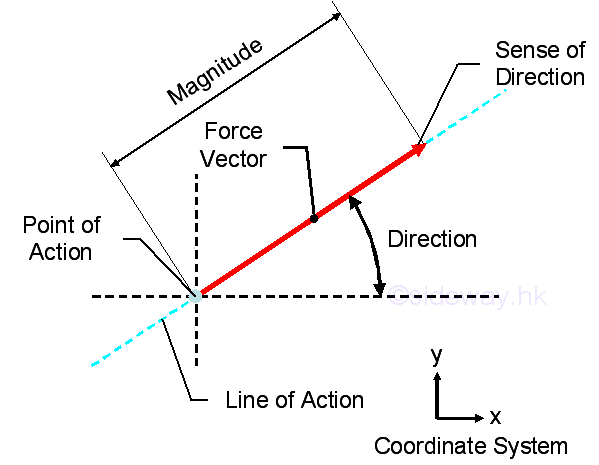1. Force of the resultant interaction at the point of interaction can either be combined or decomposed.
http://output.to/sideway/default.asp?qno=110400005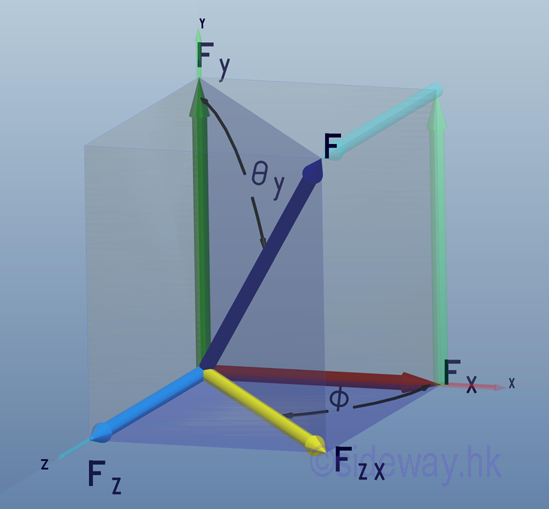2. The point of interaction is in static equilibrium when the resultant forces by Newton's first law of motion, acting on it is equal to zero.
http://www.output.to/sideway/default.asp?qno=110400006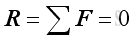3. Force in a plane is just a special case of force in space with one rectangular force vector component equal to zero.
http://www.output.to/sideway/default.asp?qno=110400005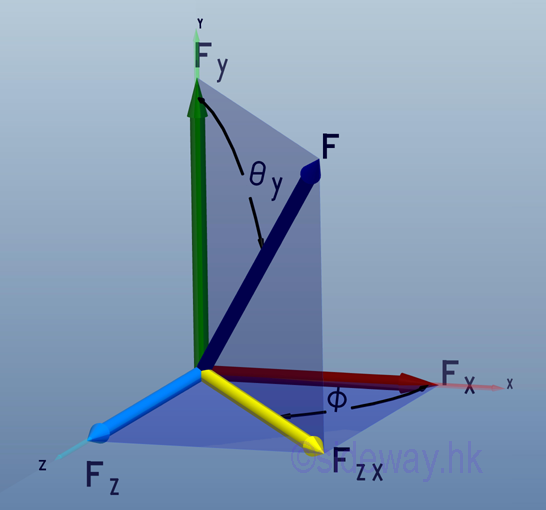4. The vector representation of a force has the advantage of manipulating both the magnitude and direction of a force at the same time trigonometrically with the help of geometry especially in plane force manipulation.
http://www.output.to/sideway/default.asp?qno=110400006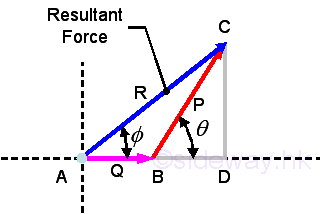5. Unit vectors, i, j, and k with unit magnitude are usually used to represent the directions of the three rectangular force vector components of a force in space after decomposition so that forces can be manipulated algebraically along the three axes of the coordinate system.
http://www.output.to/sideway/default.asp?qno=110400005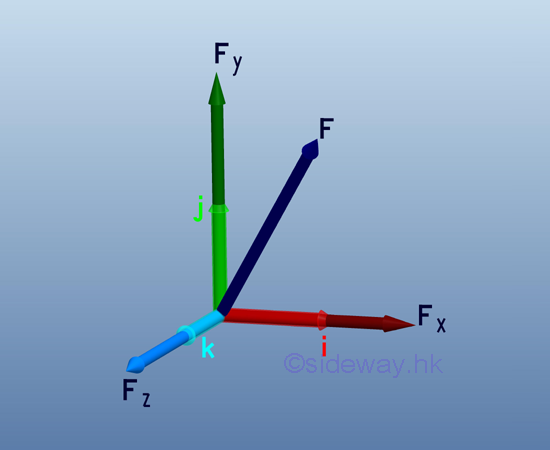6. Direction cosines of a force which is equal to the cosines of θx, θy, and θz, are just the magnitude of the three rectangular force vector components of the unit vector λ along the force vector F and can be used to tackle problems with angles between the force vector and coordinate axes are known.
http://www.output.to/sideway/default.asp?qno=110400005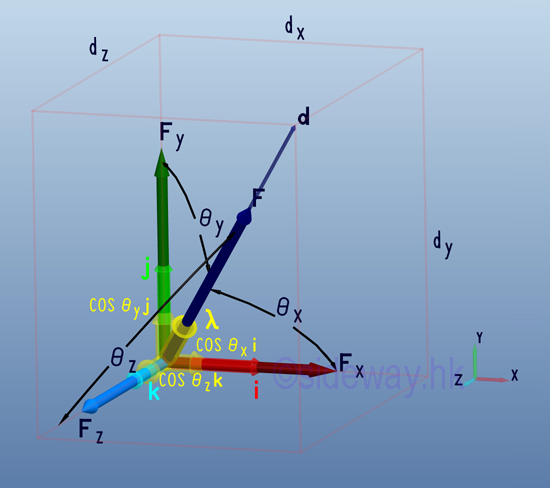3. Free Body Diagram

Free body diagram is one of the common techniques used in solving engineering mechanics statics problems by making use of the Newton's third law to isolate the interested area from the other parts of the whole system through the disconnection of the interaction between the interested area and the whole system at the idealized connection points.
http://www.output.to/sideway/default.asp?qno=110100009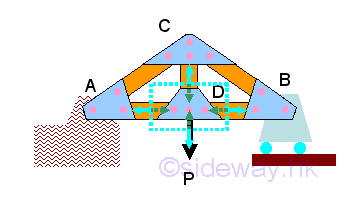4. Moment of a force is the concept used to quantify the tendency to rotate an object about a reference point as the centre of moment when forces acting on the object are non-concurrent forces and is defined as product of force F along a line of action and perpendicular distance d as lever arm of rotation between line of action and point of reference, i.e. M=Fd=Frsinθ.
http://www.output.to/sideway/default.asp?qno=110100009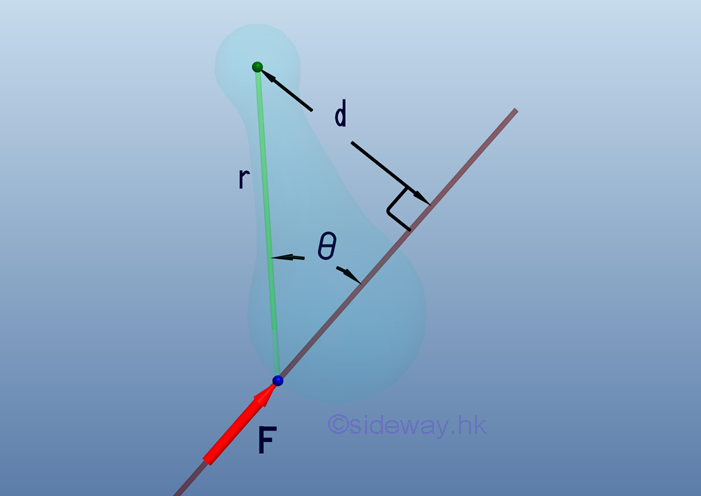1. A moment vector following the right hand rule is usually used to represent the sense and axis of moment at the interested point for the additional effect due to a force acting on an object so that concepts of motion along the line of force and the tendency to rotate about a point can be handled separately.
http://www.output.to/sideway/default.asp?qno=110100009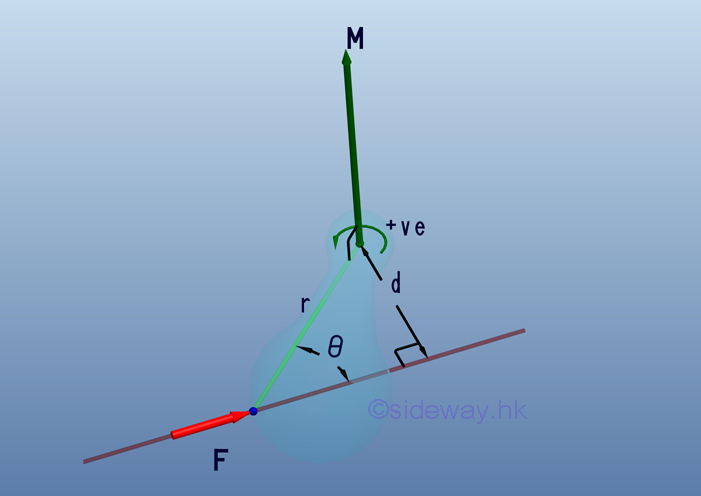2. When moment is treated as a vector quantity, moment vectors itself can be combined or decomposed through vector manipulation similar to force vector manipulation and the moment vector of a force about a point can also be obtained by the cross product operation of the position vector 𝐫 and the force vector 𝐅; i.e. 𝐌=𝐫⨯𝐅 or can be expressed in the determinant form.
http://www.output.to/sideway/default.asp?qno=110600002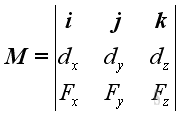3. Moment about point is tendency to rotate about axis along moment vector which is not always aligned with allowed rotation axis in practice, the moment about specified axis can be obtained by scalar product of unit vector 𝛌 along the specified axis passing through point O and moment 𝐌 of force 𝐅 about point O, i.e. 𝑀=𝛌⋅𝐌=𝛌⋅(𝐫⨯𝐅). or can be expressed in determinant form.
http://www.output.to/sideway/default.asp?qno=110600003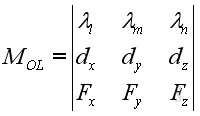5. To eliminate limitations on using moment vector 𝐌 in static analysis, force couple concept is introduced such that moment effect due to force 𝐅 at point O is transformed into a free force couple vector of same magnitude and direction as moment vector but independent of force 𝐅 and point O since net force of force couple 𝐅₁𝐅₂ is zero, after translating force 𝐅 to point O.
http://www.output.to/sideway/default.asp?qno=1106000056. By the moment vector 𝐌 of force couple concept, system of forces acting on an object about the interested point O can be transformed into a resultant force vector 𝐑 by translating all forces 𝐅ᵢ to the interested point with moment effect is replaced by a free moment vector accordingly and a resultant moment vector 𝐌ₒ by vector addition of all free moment vectors 𝐌ᵢ.
http://www.output.to/sideway/default.asp?qno=1107000137. An object is therefore in static equilibrium state only when both translational motion due to resultant force 𝐑 and rotational motion due to resultant moment 𝐌 are equal to zero.
http://www.output.to/sideway/default.asp?qno=1202000591. By making use of free body diagram technique, an object can be any part of a mechanical system and since only six (three) equilibrium equations can be obtained from a rigid body in three (two) dimension no more than six (three) unknowns can be determined by the system of equilibrium equations and vector operation is the more convenient way to determine unknowns.
http://www.output.to/sideway/default.asp?qno=120200067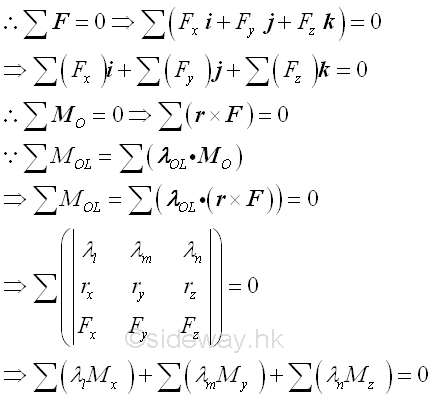8. Truss is an engineering structure with members in the truss are designed for supporting only axial loading of tensile and compressive force while bending moment is negligible and all jointed truss members can be considered as separated truss members with frictionless hinge pin joint as loads are always supported by the rigid three member triangular like structure.
http://www.output.to/sideway/default.asp?qno=1202000691. Besides the forces in truss, the other two main concerns in truss design are stability and determinacy such that any unstable member arrangement and over rigid or constrained design are eliminated in the design.
http://www.output.to/sideway/default.asp?qno=1202000712. Forces in trusses can be determined by method of joints through setting up two/three equilibrium equations for each point together with three/six static equilibrium equations to determine unknown reactions or by constructing force vector diagram with known forces and structure dimensions and therefore method of joints always start with joint with two/three unknown forces.
http://www.output.to/sideway/default.asp?qno=120200072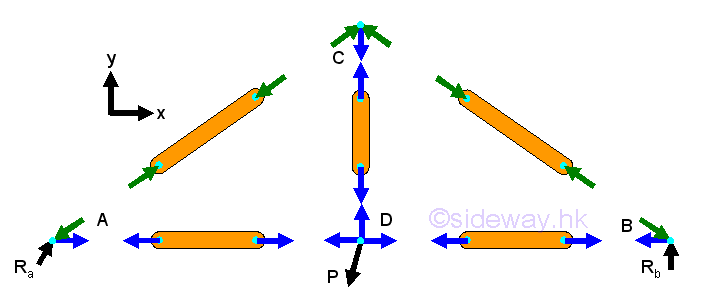3. Forces in trusses can also be determined by method of sections through setting up three/six equilibrium equations for each virtual isolated section of truss with unlimited shape as a rigid body together with three/six static equilibrium equations to determine all unknown reactions and force vector diagram at joint may provide additional equilibrium equations also.
http://www.output.to/sideway/default.asp?qno=1202000744. Frame is structure to support load similar to truss but with at least one multiple force member of more than two forces and all forces acting on the member are not along the direction of member axis and therefore unlike truss, although forces acting upon frame member can be determined similar to force analysis in truss, but unlike truss, moment should be considered also.
http://www.output.to/sideway/default.asp?qno=1203000105. Machine is mechanical structure similar to frame but with moving component designed to transmit and modify forces and therefore a machine is usually a collapsible frame, but forces acting upon machine component can be determined similar to force analysis in frame member with the consideration of force and moment for each rigid component part.
http://www.output.to/sideway/default.asp?qno=1203000179. Besides the concentrated force which is represented by a force vector, the most common forces in practical problems are distributed forces which are either body forces acting over a volume or surface forces acting over an area and in general these distributed forces can also be represented by a concentrated force through system of forces transformation.
http://www.output.to/sideway/default.asp?qno=120300021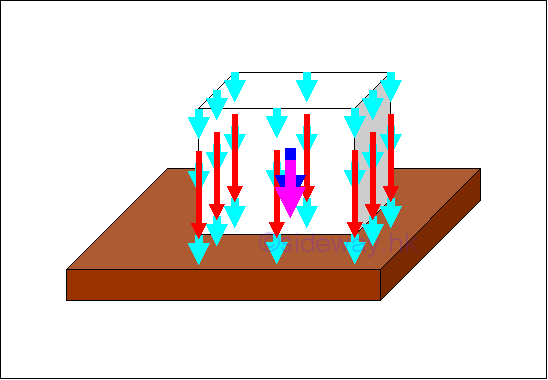1. Body Forces

Weight of a rigid object which can be considered as distributed body forces 𝐖ᵢ of infinitesimal element over a volume, can be represented by a single force 𝐖 through system of forces transformation and determined by equating the two systems of forces where the location of force is called centre of gravity which is same as the centroid of volume for a homogeneous body.
http://www.output.to/sideway/default.asp?qno=120600009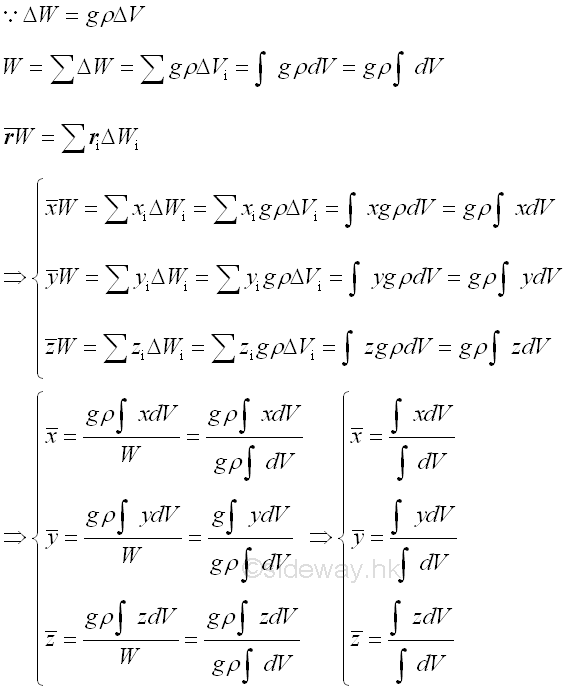2. Surface Forces

Similarly body dead load acting on a surface can be considered as distributed surface forces 𝐖ᵢ of infinitesimal element over surface normally which can be represented by a single force 𝐖 through system of forces transformation and determined by equating two systems of forces where location of force for a homogeneous distributed load depending on shape of load only.
http://www.output.to/sideway/default.asp?qno=12070000510. Besides distributed normal or inclined surface forces, friction force due to the relative lateral motion of two contact surfaces is a common distributed tangential surface force found in practical engineering problems and maximum static friction force which is proportional to the normal applied force can be developed when two surfaces are impending.
http://www.output.to/sideway/default.asp?qno=120700007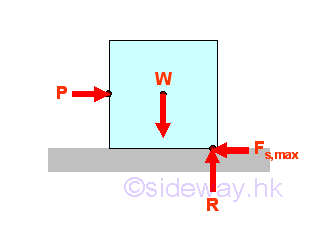11. When a member of an rigid body is in static equilibrium, the internal forces in the member tending to resist those external forces should be in equilibrium similar to the structure analysis by section also but the internal force should be represented by a force-couple system instead of a force vector system since shear forces and bending moments are usually involved.
http://www.output.to/sideway/default.asp?qno=120800020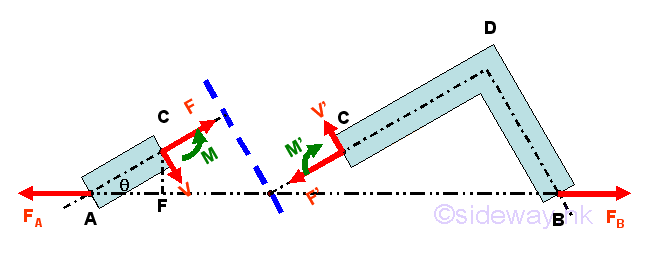12. In general, second moment of a body about a reference is the summation of moment of distributed forces of intensity proportional to the distance between force and reference over the body relating to the square or second order of distance and shape of the body only while first moment of a body about a reference is relating to the distance and shape of the body only.
http://www.output.to/sideway/default.asp?qno=12100000113. When a body in static equilibrium, since all forces are in equilibrium and the resultant force on any particle is equal to zero, the work done by the resultant force is equal to zero also and therefore the work done on the particle for any virtual displacement δs is equal to zero as the resultant force acting on a particle is zero.
http://www.output.to/sideway/default.asp?qno=121100089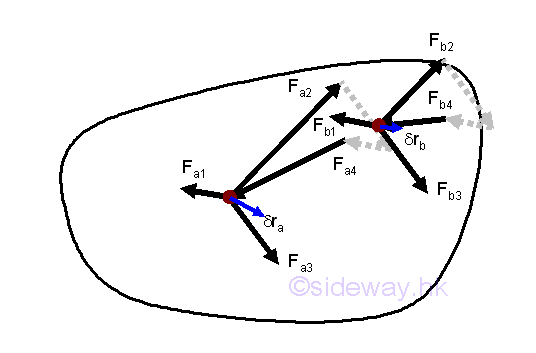ID: 130800173 Last Updated: 8/20/2013 Revision: 0 Ref:References

1. I.C. Jong; B.G. rogers, 1991, Engineering Mechanics: Statics and Dynamics
2. F.P. Beer; E.R. Johnston,Jr.; E.R. Eisenberg, 2004, Vector Mechanics for Engineers: StaticsHome 5

Management

HBR 3

Information

Recreation

Culture

Chinese 1097

English 339

Computer

Hardware 224

Software

Application 213

Latex 52

Manim 204

KB 1

Numeric 19

Programming

Web 289

Unicode 504

HTML 66

CSS 65

SVG 46

ASP.NET 270

OS 429

Python 72

Knowledge

Mathematics

Algebra 84

Geometry 33

Calculus 67

Engineering

Mechanical

Rigid Bodies

Statics 92

Dynamics 37

Control

Natural Sciences

Electric 27